The Apollo Gauss Cannon
April 2014

# 3.  Conception and construction

This page will cover the practical side of the building of Apollo. We will apply the formulae derived in the previous page and see how this coilgun was actually constructed. Regarding electrical components, I will give the references and datasheets only for the "special" and most relevant parts ; unless specified, things like diodes or bleeder resistors are standard-type.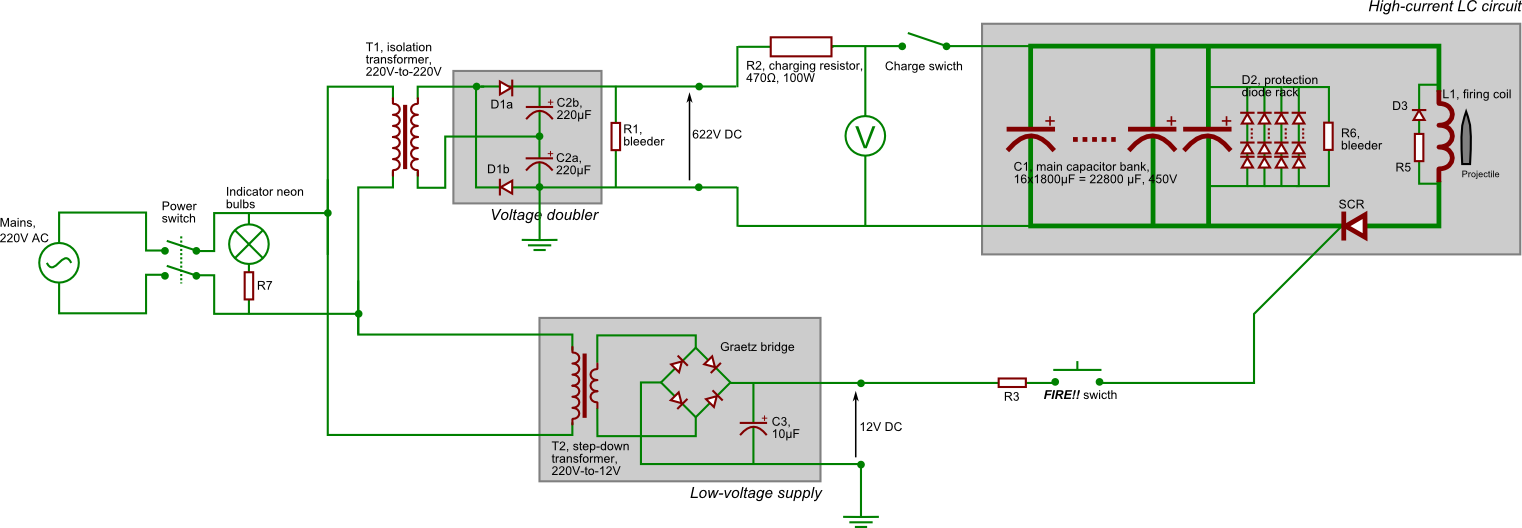Figure 3.1 — Complete electrical schematic of the Apollo Gauss cannon.

## 3.1   Main LC circuit

### 3.1.1   Capacitor bank

The capacitor bank is, in a way, the most important component of the coilgun, as it stores the energy that will be released upon firing. The energy of a shot, which is primarily determined from the energy that can be stored in the capacitors, is the main design driver of a coilgun, since, as we'll see, the characteristics of many components (such as the inductance of the firing coil) will depend on it.

Apollo's capacitor bank is made of sixteen capacitors connected in parallel, with each one having a capacity of 1800 µF and a tolerance of 450 V. We thus have a total of :

Ctot = 28.8 mF,

Tolerance = 450 V.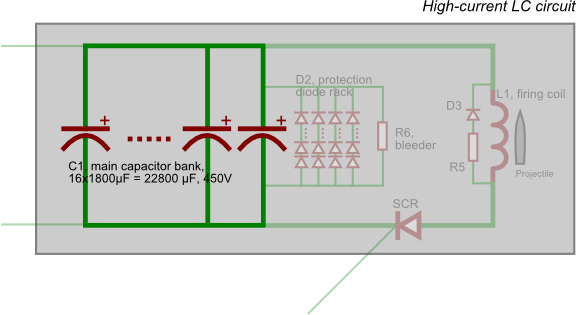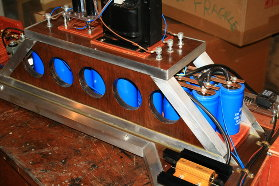Figure 3.2 — Position on schematic (highlighted) and photograph of the main capacitor bank of Apollo.

#### Power considerations

As we saw in the introductory section of the previous page, the energy stored in a capacitor of capacity C charged under a difference of potential V is given by E = CV2/2. Note that energy grows quadratically with voltage, which is one reason to favour capacitors with a large voltage tolerance. Using the values given above, we see that Apollo's capacitor can store up to

Etot = 2.916 kJ.

While the previous formula gives the maximal energy available, a very small fraction of it will actually be converted into kinetic energy of the projectile when the coilgun is fired. Most of the losses occur due to the relatively weak magnetic coupling between the firing coil and the projectile, but other effects such as imperfect discharge time, friction and ohmic losses can be important too. For most home-made coilguns, efficiency ranges from 0.5 to 5 percent. We will need the expected kinetic energy of the projectile to compute the ideal inductance of the firing coil below. Let us be optimistic and consider an efficiency of 5%. The actual (expected) energy of a shot will thus be taken to be

Eeff = 145.8 J.(3.1)

#### Construction

Electrolytic capacitors are practically the only type of capacitors able to deliver the kind of capacity and tolerance needed for a Gauss cannon while still remaining easily available. The capacitors used on Apollo are Nippon Chemi-con U36D capacitors (not in production any more, but the U37X series is similar, see datasheet).

Having a very small resistance in the firing circuit is of paramount importance, as this was thoroughly explained in the theory page. Good coilgun capacitors should thus have the lowest equivalent series resistance possible. To further limit the resistive losses, Apollo's capacitors were connected together via thick copper bars, which will have a very high current capacity. These were made by folding copper tubing twice and drilling holes at the emplacements of the capacitors' contacts. They can be see on photograph below (fig. 3.3), taken during early stages of Apollo's construction.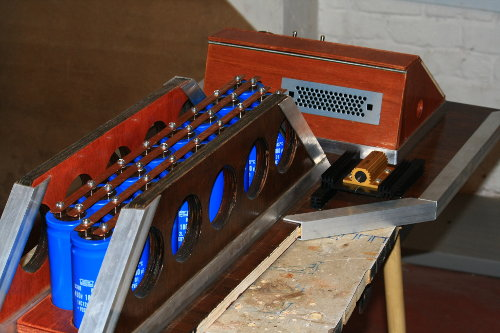Figure 3.3 — Photograph of Apollo's capacitor rack, before the power supply platform was added on the top of it. The copper bar which connects all the capacitors in parallel can clearly be seen.

### 3.1.2   Projectile

The main design driver for the firing coil is actually the projectile that it be asked to fire, we shall thus cover this point before moving on to the coil design section. The length and diameter of the coil will indeed be chosen to optimise the performances with a projectiles of pre-determined dimensions ; therefore a coil will, in general, not be able to efficiently launch a wide type of projectiles. The choice of that projectile is up to the builder's preferences. Most coilguns will fire small, bullet-like projectiles, but for Apollo I rather opted for a much larger and heavier type. The projectile used has a cylindrical shape, with a diameter of D = 2.9cm, a length of l = 9.7cm, and a weight of about 351 g.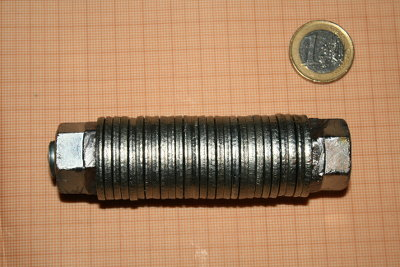Figure 3.4 — Photograph of the projectile with a 1€ coin for scale.

The material used should have a magnetic permeability as high as possible. Steel fares relatively well with a permeability of about 100 H/m, but transformer core-grade iron can reach values in the thousands. The choice of steel for Apollo's projectile was motivated more by convenience than performance, but I plan to try out a projectile made of a much better material in the future. The projectile shouldn't be too smooth in order not to allow for too large Foucault currents, which would dissipate energy that would otherwise be used to accelerate the projectile. A segmented design such as the one used here provides some minimal protection against this.

Lastly, let us calculate the speed that the projectile is expected to acquire using the effective energy Eeff given at eq. (3.1). Using v = (2E/m)1/2, then setting, in our case, E = 145.8 J and m = 351 g, we obtain :

vmax = 28.9 m/s,(3.2)

which is about 100 km/h (!). This is likely to be quite an optimistic estimate since we assumed an efficiency of 5%, which is probably a bit too high (and, indeed, I was only able to reach a speed of 80 km/h as we'll see in the next page), but will be a useful guide nevertheless.

I have also made a lighter and smaller projectile (292g, 8.2cm) to see with which one the coil is the most efficient (see next page), but generally I don't use it otherwise.

### 3.1.3   Firing coil

The firing coil will generate the magnetic field that will propel the projectile through the cannon's barrel. It also provides the inductive part of the LC firing circuit. General guideline for the firing coils are :

• A length equal to about 1.5 times the length of the projectile.
• A minor diameter as close as possible as the diameter of the projectile, to avoid "wasting" magnetic field in the inter-space between these two elements.
• As for the rest of the firing circuit, a very high current capacity and a resistance as small as possible. This is made possible with the use of large cross-section conductors and good contacts.

The coil of Apollo has a minor diameter d = 3.3 cm, a major diameter D = 7 cm and a length L = 13 cm. The wire used is a copper wire with a 10mm2 cross-section and a 1mm-thick PVC insulation.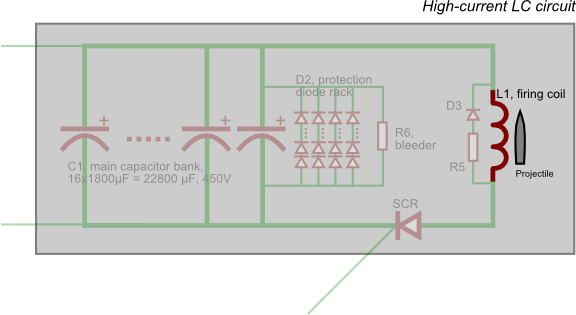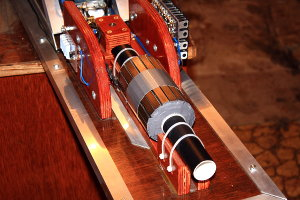Figure 3.5 — Position on schematic (highlighted) and photograph of the firing coil of Apollo.

#### Inductance

The proper inductance of the coil will be determined as the inductance needed so that the discharge time is equal to the time the projectile will take to travel to the middle of the coil. Indeed, once the projectile goes past this point, any magnetic field that would remain would just tend to attract it back into the coil, thus lowering the cannon's performances. To calculate that time, we will assume that acceleration is constant and that no friction force is present. The result we'll obtain with such assumptions will be quite crude, but will provide an useful estimate of the needed inductance.

Given the final speed vmax determined at eq. (3.2), we are able to calculate the crossing time using elementary mechanics. The initial speed of the projectile is in this case zero, and we set its initial position to be zeto as well. Since acceleration is supposed to be constant, our equations of motion take a very simple form : x(t) = at2/2, and v(t) = at. Cancelling the unknown acceleration a between the equations, we find that the time t* needed for the projectile to reach the speed vmax will be given by

t* = 2x*/vmax,

where x* is the distance crossed during that time. In principle, we should set this distance to be half the coil length L, but in practice, the projectile's own length and its starting position will make that distance a little longer. In this back-of-the-envelope calculation, I used three quarters of the coil length instead, which thus gives us t* = 1.5L/vmax. Using the corresponding numerical values, we find

t* = 5.6 ms.(3.3)

We can now determine the "ideal" inductance for our cannon. If we make the assumption we have a LC circuit, then we need to adjust the inductance so that the period T of oscillations is equal to 2t*. Indeed, a period engulfs a complete oscillation while we only want half that to accelerate our projectile (the other half is supposed to be cancelled by the diode system we described on the previous page). Using ω = 2π/T = (LC)1/2, we find that T = 2π(LC)1/2 and consequently t* = π(LC)1/2. Solving for L, one finds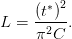When replacing t* by its corresponding value given at eq. (3.3) and C by the capacity of Apollo's capacitors, 28.8 mF, we find :

L = 111 µH.

This is of course a very small value, which will be very difficult to measure/realise. There exist empirical formulae that give the inductance based on a few physical parameters (see for instance B. Hansen's applet). In practice, we will try to get a coil whose inductance is the same order of magnitude as the desired inductance and will achieve the best discharge timing by varying the initial position of the projectile within the coil. Nevertheless, the inductance of the coil was measured using a cheap LRC bridge, and an inductance of (0.2 ± 0.1) mH was detected. The error interval is very large due to bridge being used near its very detection threshold.

#### Magnetic shield

A topic we haven't yet developed is magnetic reluctance. Intuitively, this is the "magnetic analogue" to the well-known electrical resistance, and is defined through an equation very similar to Ohm's law. Indeed, R the reluctance of a magnetic circuit, we have

R = F/Φ,

where F is the "magnetomotive force", the magnetic equivalent of the electromotive force and Φ the magnetic flux. For a homogeneous circuit, the reluctance will be given in the most general case by

R = l/(μs),

where l is the length of the circuit, μ its magnetic permeability, and s its cross-section. From that equation we also deduce that the SI units for reluctance are inverse henrys H-1.

It would seem that that for situations such as those encountered on coilguns, the main limitation to energy transfer to the projectile is magnetic saturation of the circuit (see e.g. B. Hansen's page) . Consequently, reducing the reluctance of the circuit will be of prime importance for good coilgun performances. This is usually achieved by encapsulating the coil, where most of the magnetic field is, around a strongly ferromagnetic material. Because the permeability of such a material will be much higher than the permeability of the air, the reluctance of the circuit will be decreased. This layer of ferromagnetic material around the coil is referred to as the magnetic shield. In practice it is composed of two parts :

• The longitudinal section, covering the long dimension of the coil.
• The end caps, covering the front and back of the coil.

Regarding the optimal dimensions of the shield, it turns out most of the reluctance of the firing coil will actually be due to the air core (through which the projectile goes), so the actual thickness of the shielding will be more or less irrelevant. Much more information can be found on here.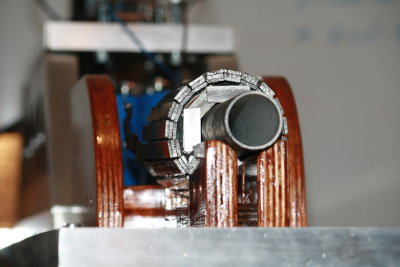Figure 3.6 — A photograph of Apollo's firing coil. The shielding is the metallic structure around the coil, which can be seen nicely here (a reinforcing resin was added later, which hides the end caps). The coil itself can be seen beneath this structure if you look carefully. Looks a bit like the CMS, doesn't it ?

The magnetic shield of the Apollo Gauss cannon was built out of sections of 3mm-thick steel bars, as it can be seen on fig. 3.6 above. Steel is definitely not the most permeable material, I used it out of convenience just like I did for the projectile. A ferrite shielding made of remains of a transformer core will no doubt be more efficient, but that option would have been difficult to apply to Apollo's very large coil. The total thickness of the shielding is about 1 cm.

#### Construction

Barrel. The gun barrel provides a guiding structure which makes sure the direction of the projectile is under control. For its construction, any rigid, diamagnetic material will do (a ferromagnetic barrel would "divert" the magnetic field into itself, leaving almost nothing for the projectile). It should be as thin as possible in order to avoid wasting space between the coil and the projectile, but must remain rigid. On Apollo, a simple 2mm-thick PVC tubing tubing was used. The inner diameter is 2.9 cm which is just enough to let the projectile pass, and its length is about 33 cm. The inside is regularly oiled to reduce friction and improve performance.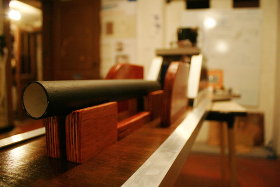Figure 3.7 — Close-up on Apollo's gun barrel, when neither the coil nor the shielding was present around it.

Coil. To limit resistance, the use of a very thick and highly-conducting wire is recommended. On Apollo, a 10mm2 cross-section copper wire with a 1mm PVC insulation was used. Three layers of windings were placed. After placing each layers the coil was consolidated using a strong adhesive tape to avoid disruption.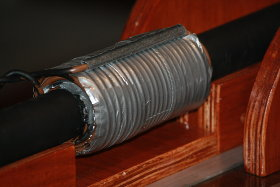Figure 3.8 — Apollo's coil assembled, upside-down on this picture.

Shielding. Both the longitudinal section and the end caps were made using the same stuff, a 3mm-thick steel bar. The sections were glued together to obtain the required shape. Once all of the shielding was placed, I drowned the assembly in a resin. There are indeed very strong compressive forces applied on the shielding when the coil is fired (the first version of the shielding was shattered during test fires, as we'll see on the next page), and simply gluing the bars onto the coil wasn't enough. This resin, essential to keep the shield together, is slightly elastic once dry, which helps relieve some strain on the parts.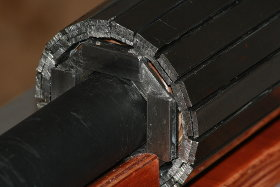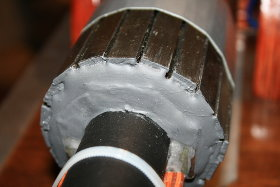Figure 3.9 — First : Longitudinal section placed. Second : End caps added. Third : Reinforcing resin added.

Assembly. The whole firing part is firmly attached to the wooden structure of the device using cable ties, as it can be seen in fig. 3.5 above. Behind the cannon lies the firing platform, on which the projectile is placed. The wooden obstacle in the centre prevents the cannon from backfiring and destroying the brittle electronics behind it (this actually happened). Finally, the wires of the coil are connected to the rest of the firing circuit via plate terminals. Note those wires are twisted to limit stray inductance.

### 3.1.4   Some remarks and figures

Let us pause for a moment and determine the peak current flowing into the cannon circuits. This will be useful to size components like the SCR and it's a pretty interesting number in its own right. We have a capacitance of 28.8 mF and an inductance around 200 µH. Moreover we will estimate the total resistance of the circuit to be 0.05 Ω, which seems like a reasonable value. The initial charge of the capacitors will be given by Q0 = C·450 V.

The behaviour of such a RLC circuit was, in fact, already analysed in the theory pages. Indeed, the voltage and current for an RLC circuit, given at figs. 2.12 a and b were generated using the numerical values given above. Additionally, all plots from section 2.2.3, where the detailed analysis of the coilgun circuits is made, were generated using numerical values compatible with Apollo.

In particular, we can see in fig. 2.12b that the inductor current is going up to more than 3400 Amps. This is a really huge number. For comparison, these kind of currents are used in electric welding machinery. The duration of that peak is very short : as we can see, a complete semiwavelength lasts only 7-10 ms when protection circuits are taken into account.

Still, whenever an electrical connection on Apollo wasn't perfectly secured, a bright, noisy spark would happen, and I would often find the said connection to have melted. This happened for instance on this connection near the SCR, where the lower screw and one of the terminals it was touching merged together.

## 3.2   Charging circuit

### 3.2.1   Power supply

The power supply provides the necessary DC voltage to build-up a charge in the capacitors, charge which will later be released when the cannon fires. We've seen in the previous section that it was advantageous to construct a Gauss cannon around high voltage-rated capacitors, and this is indeed what was done on the Apollo Gauss cannon, whose capacitors have a rating of 450V. In Europe the current from the main is 220V AC, so in our case, we'll need a circuit that is able to step up this 220V AC into (at least) 450V DC.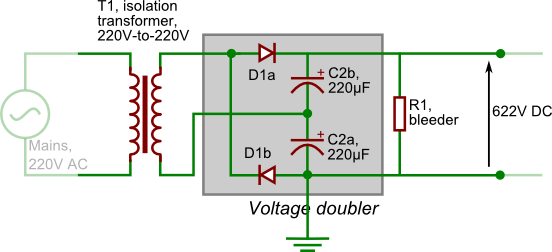Figure 3.10 — Position on schematic (highlighted) and photograph of the power supply of Apollo. On the photograph, you can see two high-voltage pins on the left.

Apollo's power supply is basically composed of a voltage doubler and of an isolation transformer. An isolation transformer is simply a 1:1 transformer used to physically separate the charging circuit from the mains for additional security. The model I used on Apollo is a 230V-to-230V Schneider Electric ABL6TS25U (see datasheet). The voltage doubler is a slightly more complex apparatus. Let us have a look at it.

#### Full-wave voltage doubler

This circuit is composed of two capacitors and two diodes, connected as shown on fig. 3.10 above. This is a very generic circuit, which can be found in any electronics textbook (see All About Circuit's entry for instance). There is no strict rule regarding the capacity that these capacitors should have. They must just be large enough so that ripples are small and do not cause too much of a variation in the output. On Apollo I used 220 μF, 400V-rated electrolytic capacitors from the Panasonic EE series (see datasheet), and charging appears to be very smooth. As per the design of the circuit, both the capacitors and the diodes should be able to withstand at least half the output voltage.

Notice that there's also a 450 kΩ bleeder resistor between the output leads, which will slowly discharge the capacitors after use. This small addition is not strictly necessary for the good functioning of the voltage doubler, but is an important security feature that ensures the device is "safe to touch" after use.

A voltage doubler will take an alternative voltage and covert it into a direct voltage which will be equal to twice the amplitude of the original AC voltage. Let's keep in mind, however, that engineers don't usually characterize alternating electric signals by their amplitude but rather by their root mean square (RMS) value, which differs by a factor of √2. Therefore, if we call VRMS the RMS value of the input AC voltage, the output voltage V will be given by 23/2VRMS. In Europe the mains voltage is 220V; so the DC voltage that our voltage doubler will be able to supply will be equal to

Voltage = 622 V

Which is more than enough to charge capacitors that can only hold 450V. We will actually have to be careful to to overcharge them, as we'll see it in the charging resistor section just below.

### 3.2.2   Charging resistor

The charging resistor, placed between the voltage doubler and the capacitors we want to charge (see R2 on the main schematic at the top or on fig. 3.11 below), provides a resistive part to the charging circuit, and thus makes it a RC circuit, which we discussion in length in the previous page. It's role is simply slow down the rise of the voltage across the capacitor, as shown by the figure 2.8, which is needed mainly for two reasons :

• An empty capacitors looks like a short-circuit from the power supply's side. Without a resistor, the brutal rise in current that would occur could be unhealthy for both the capacitors and the power supply (here, the voltage doubler). Moreover, this will also result in high voltages being generated which could be dangerous as well.
• As shown above, the power supply generated a direct tension of 622V, while our capacitors can only sustain a voltage of 450V. Slowing down the charging will thus allow us to monitor the rise of the voltage across the capacitor and stop it when that voltage reaches 450V. If we were to let the voltage increase past this value, the capacitors could be destroyed.

The value of that resistance must give a good balance between charging time and instantaneous power : if the resistance is too high, the charging time is likely to become impractical, whereas if it is too low, the currents involved will be very high and we run into the two issues we just mentioned. The resistor I used on Apollo is a 470 Ω, rated for 100 W, from the Welwyn WH series (see datasheet). It is mounted on a heatsink for better heat dissipation, as it can be seen on fig. 3.11 below. As we'll see in a moment, this value provides, in this particular case, a good trade-off between charging time and power.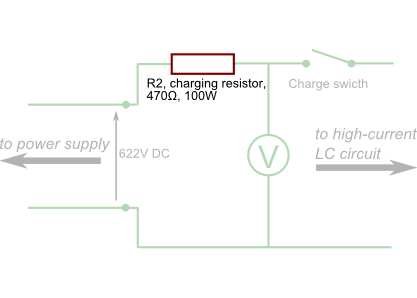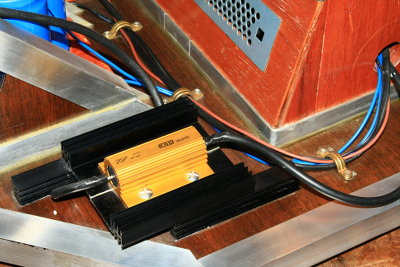Figure 3.11 — Position on schematic (highlighted) and photograph of the charging resistor of Apollo, fixed on an extra heatsink.

For how long do we need to charge the capacitors to their maximum voltage ? Formula 2.9 tells the voltage across the capacitor with respect to time. Isolating the time t in it, and replacing V0, R and C by their value in Apollo's circuits, i.e. respectively 622 V, 470 Ω and 28.8 mF, we find that tCharge such as V(tCharge) = 450 V is equal to

tCharge = 17.40 s

In other words, it takes about 17 seconds to reach 450V in the capacitors with the circuit used. We will thus have to disconnect the capacitor rack from the charging circuit after about 17 seconds. In practice, we use a voltmeter to monitor the rise and perform the disconnection based on its readings (see Control panel below). Regarding power dissipation, the heating of the resistor after a full charging cycle is very acceptable.

## 3.3   Trigger circuit

What I call the "trigger circuit" consists of the SCR, its gate power supply, and the firing button line. On the main schematic at fig. 3.1 above, it is the whole lower part of the circuit.

### 3.3.1   Silicon-controlled rectifier

The silicon-controlled rectifier is surely the least common component of a Gauss cannon. On the theory page we explained how such a device worked and presented some elements of its operation. On a coilgun, its role will be to close the main LC circuit when the user pushes the firing button, so it basically acts like a very high current capacity switch. Indeed, we saw in section 3.1.4 that the kind of currents the LC circuit has to deal with range in the kilo-ampères, and no standard (mechanical) switch could possibly handle that safely. Hence the need for an electrically-controlled switch. The SCR seems to be the most widespread option for coilguns but other kinds of power electronics such as IGBTs can be used.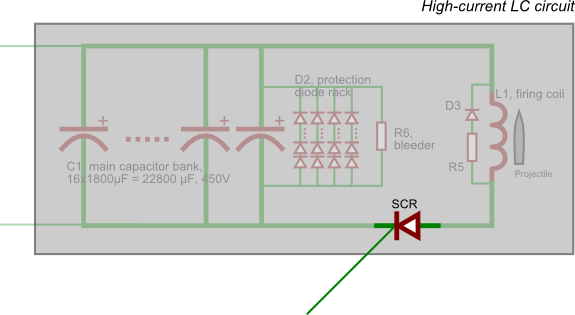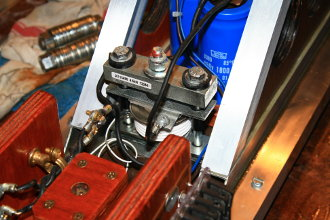Figure 3.12 — Position on schematic (highlighted) and photograph of the SCR of Apollo.

The model used for Apollo is an Infineon T1500N08TOF VT Phase Control Thyristor (see datasheet), which has a 800 V tolerance and a peak current capacity of more than 33 kA. This is far more than enough for Apollo, but initially I preferred to play it safe instead of frying something. However, I now believe that a smaller model such as the T920N06TOF (600V, 12 kA, see datasheet) would have most probably been enough.

#### Clamp assembly

These "hockey-puck" shaped rectifiers need to be clamped with considerable force (several kN) in order to work. Indeed, the conductive surfaces of these devices do not normally touch unless they are compressed that way. To achieve this, the use of purpose-made clamps with predetermined maximal forces is recommended ; more information on SCR mounting can be found in this document. Note that this mounting is usually achieved with massive heatsinks when SCRs are normally used in continuous mode, where a great amount of thermal energy needs to be dissipated, but for Gauss cannon we only use the device to control very short pulses, and this won't generate a lot of heat.

The clamp used for Apollo's SCR is rated for 4.5 kN. Additionally, protruding aluminium bars were placed within the clamp assembly in order to place wire terminals. This allows to easily connect the SCR to the rest of the cannon.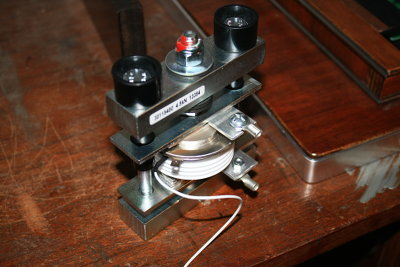Figure 3.13 — Apollo's SCR in its clamp. The thin white wire is the gate connection while the two terminals screwed on aluminium plate connect to both poles of the device.

### 3.3.2   SCR gate firing circuit

To make the SCR conductive, and thus fire the whole cannon, a small DC voltage has to be applied to the SCR gate. The circuit providing that controlling voltage on Apollo is quite simple. First, a 220V-to-12V step-down transformer produces a low-voltage AC signal (marked as T2 is figs. 3.1 and 3.14). Then, this waveform passes through a Graetz bridge rectifier, followed by a small 10 µF electrolytic capacitor (C3) to cancel out the ripples. This is a very commonplace kind of circuit, you can read more about it here. Finally, a 110 Ω current-limiting resistor (R3) and a pushbutton switch delivers the small pulse to the SCR. The button itself lies on the control panel, which we will talk about in the last section.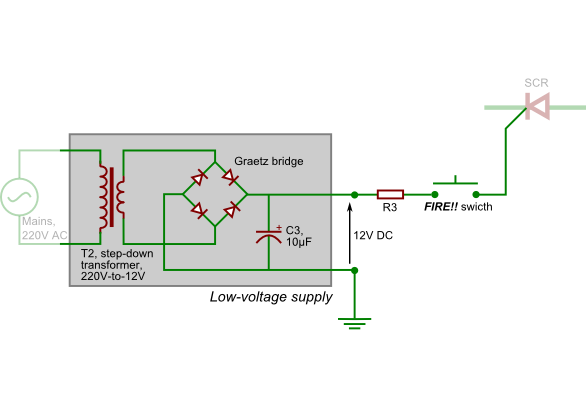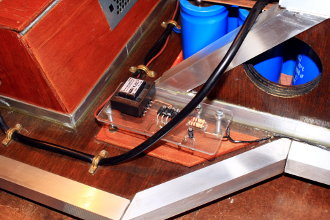Figure 3.14 — Position on schematic (highlighted) and photograph of the SCR of Apollo. The last picture shows the circuit upside down, where all handmade connections can be seen.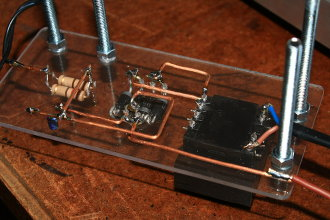Figure 3.15 — The circuit upside down, before it was mounted, where all handmade connections can be seen.

## 3.4   Protection system

### 3.2.1   Capacitor branch

The capacitor protection branch ensures that capacitors are not subject to a dangerous level of reverse-voltage. Indeed, electrolytic capacitors (the type used in most coilguns) do not cope well under such situations, which can lead to the explosion of the component. In its simplest form, this protection system consists of a single diode, but as we saw in the theory page, this causes a dramatic lengthening of the discharge time, which, in turn, decreases the cannon performances quite awfully. We also reviewed in great lengths what other solutions were available and in which way the behaviour of the cannon was affected. The two solutions retained were :

• Diode with resistor. See fig. 2.24 to visualise the effect on voltage and current. Note that the plot was obtained using Apollo's own circuit values for L, C, etc.
• Multiple diodes in series to increase forward drop. See fig. 2.25 for voltage and current. Same remark.

As we can see from fig. 2.24, the diode with resistor solution seems to be working pretty well : with a series resistance Rd = 0.043 Ω, we reduce the discharge time down to only 11 ms while the reverse voltage goes only up to -30 V for less than 5 ms. However, I wrote that little simulation only after building my Gauss cannon, and at the time I preferred playing it safe and using diodes in series with a moderate total forward drop. I plan to change the protection system at some point in the future to implement the more effective diode with resistor-type protection circuit.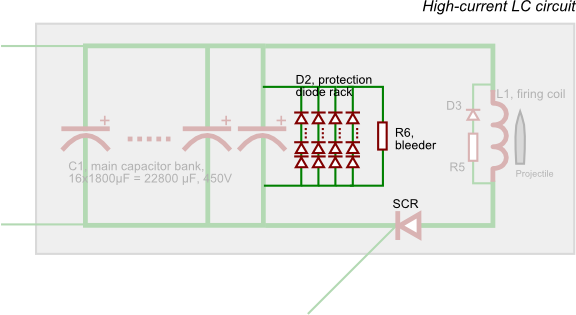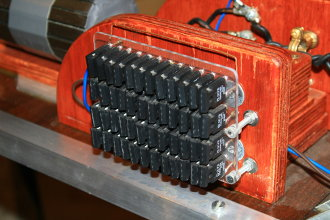Figure 3.16 — Position on schematic (highlighted) and photograph of the diode network protecting Apollo's capacitor rack from harmful reverse-charging.

I thus went with the diodes in series paradigm, as you can see in fig. 3.16 above. Two versions have been used on Apollo, as we'll see below. We will be using the results of the circuit simulation several times on the following section, I have thus re-linked the script from page 2 here for convenience (link on the right). Also, not really related to protecting the capacitors, but I included a 450 kΩ bleeder resistor (R6) in the firing circuit as well. It will slowly discharge the capacitors once the device is off-line. This makes the device a little safer.

#### Version 1.

In its first implementation, that branch consisted only of twelve humble UF3007 diodes (datasheet), each having a forward drop of 1.7 V and a surge current rating of 125 A. The branch thus provided a total forward drop of 12×1.7 V = 20.4 V, which is a perfectly safe level as the circuit simulation shows.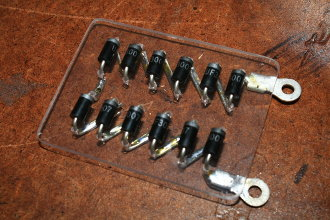Figure 3.17 — Position on schematic (highlighted) and photograph of the diode network protecting Apollo's capacitor rack from harmful reverse-charging.

The current rating was, however, largely insufficient. According to the simulation, the current flowing into the circuit when the diodes kick in is about 2500 Amps (value is noted "JRLC[tswitch]" in the notebook) ; there was thus no way the diode used could have withstood this, even taking into account the very short duration of the peak. And indeed, this string of diodes was destroyed (among many other things) during the first test-fire of Apollo. There were no visible burn on the components, but the junction was conducting both ways after the test, which clearly shows the diodes were fried. A circuit with a much larger peak current rating was needed.

#### Version 2.

A more capable, and much more expansive, circuit was thus devised. The second version uses the much sturdier, German-made Ixys DSEI60-06A diode (datasheet), which has a forward drop of 1.8 V and a peak current rating of 550 A. Fourty-eight (!) such diodes were used, arranged in four rows of twelve. The total network thus has a forward drop of 12×1.8 V = 21.6 V and can withstand peaks of 4×550 A = 2200 A. With a drop of 21.6 V, the circuit simulation shows that the peak current the branch will have to withstand is 2300 Amps. This is a bit more than what the branch is supposed to tolerate, but I'm hoping that the very short time of discharge should counteract that risk.

It would seem that it does. Apollo has been fired a few dozen times and the circuit is still standing and working. Below are a couple of pictures of it during construction.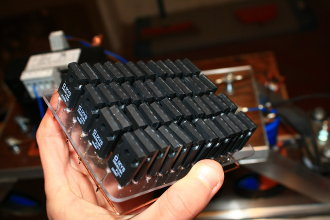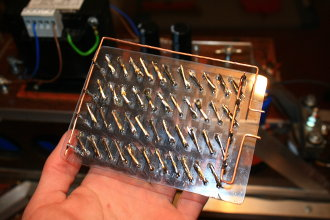Figure 3.18 — Second version of the capacitor protection circuit of Apollo during construction.

### 3.2.2   Inductor branch

The inductor protection system is far less critical than the one around the capacitor. This circuit helps protect the inductor and its surrounding from an inductive kick-back, a sharp rise in tension arising from brutal current changes (a direct consequence of Lenz' law, see here for a visualisation). Since we're using a lot of semiconductors with step-like responses dealing with huge currents, it is certainly safer to have a small protection around the inductor. This branch will typically consist of a diode with a resistance of a few ohms in series. Because of this added resistance, this branch will have almost no effect on the overall LC circuit behaviour. Indeed, the firing circuit has a resistance of a few centiohms at most, which is orders of magnitude smaller than the resistance of this protection branch.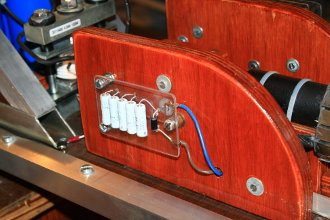Figure 3.19 — Position on schematic (highlighted) and photograph of the small protection branch of Apollo's firing inductor, which helps prevent inductive kickback.

On Apollo, a single UF3007 was used along with five 1Ω, 5W resistors connected in series (which are actually left-overs from the HV circuit of my ZM1042 clock). No problems are to be reported so far.

## 3.5   Control panel

Operating a coilgun will be much easier if all readings and manuals commands are gathered at the same place. It is also much safer, as you won't have to walk around the cannon when loaded/charged to activate and deactivate the relevant switches. On Apollo, the panel was placed at the very end of the device, and contains the following items (clockwise, starting from top-left, see fig. 3.20 below) :

• The voltmeter reading the tension in the capacitors, labelled (V) on fig. 3.1 above. A cool vintage-looking analogue model was used.
• The "Power switch", which puts the cannon, and more specifically the power supply, under tension.
• The "Charge switch", which connects the capacitors to the power supply, and thus allows them to be charged. Must be switched back off before firing the cannon. (Note that a two-switch architecture such as this one makes the cannon safer to operate).
• The red pushbutton, adequately labelled "FIRE!! switch", connects the SCR to the low-voltage supply, thus firing the projectile. What else are big red scary buttons for ?
• A brass plate bearing the name of the year of the cannon.
• A group of red neon indicators that warns the device is under tension.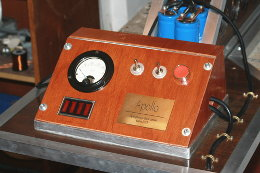Figure 3.20 — First : The control panel of Apollo. Second : The control panel open, showing all connection (the one on the right is the common ground). Third : A general top-view of the rear of the cannon.

That's it for the construction details ! The next page contains details of some notable test-fires of Apollo as well as an efficiency analysis. (Unfortunately I haven't written it yet. But I'll do it soon.)